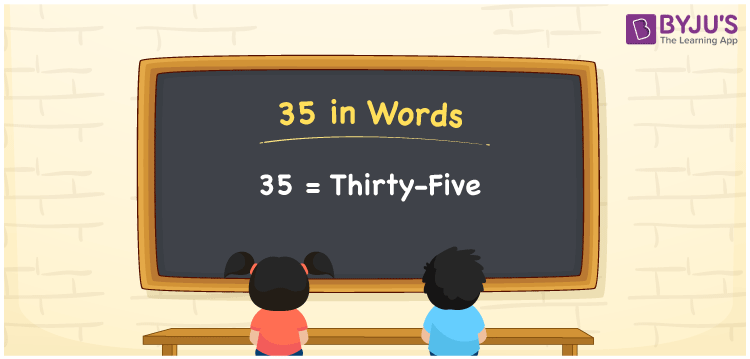# 35 in Words

Thirty-five is the number name for the numerical 35. The place values such as ones, tens, hundreds, and so on are often used to express the number name of 35. As a result, the place value table is useful for writing the number 35 in English. For example, if you spent Rs. 35 for chocolate, you can write “I spent Rs. Thirty-five on a chocolate”. Here, we will look at how to spell 35 in English words and how to write the numerical name 35 with a complete explanation.

 35 in Words: Thirty-five Thirty-five in Numerical Form: 35

## 35 in English Words## How to Write 35 in Words?

Determine each digit’s place value to write the number 35 in words. Below is a place value chart for 35:

 Tens Ones 3 5

Thus, the expanded form of the number 35 looks like this:

= 3 × Ten + 5 × One

= 3 × 10 + 5 × 1

= 30 + 5

= 35

= Thirty-five

Hence, 35 in words is Thirty-five.

The natural number 35 is found after 34 but just before 36.

35 in words – Thirty-five

Is 35 an odd number? – Yes

Is 35 an even number? – No

Is 35 a perfect square number? – No

Is 35 a perfect cube number? – No

Is 35 a prime number? – No

Is 35 a composite number? – Yes

## Frequently Asked Questions on 35 in Words

Q1

### Write 35 in words.

35 in words is thirty-five.

Q2

### Simplify 30 + 5, and express in words.

Simplifying 30 + 5, we get 35. Hence, 35 in words is thirty-five.

Q3

### How to write thirty-five in numbers?

Thirty-five in numbers is 35.